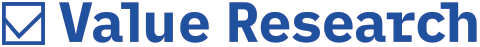# Sharpe Ratio & Beta

##### I have been investing in mutual funds and benefiting from it. I want to know about some technical terms. Please explain what Sharpe ratio and beta are.-Shveta Sinha

Beta is a statistical tool, which gives you an idea of how a fund will move in relation to the market. In other words, it is a statistical measure that shows how sensitive a fund is to market moves.

Sharpe Ratio: The Sharpe ratio is a single number which represents both the risk, and return inherent in a fund. As is widely accepted, high returns are generally associated with a high degree of volatility. The Sharpe ratio represents the trade off between risk and returns. At the same time, it also factors in the desire to generate returns, which are higher than risk-free returns.

Mathematically, the Sharpe ratio is the returns generated over the risk-free rate, per unit of risk. Risk in this case is taken to be the fund's standard deviation. A higher Sharpe ratio is therefore better as it represents a higher return generated per unit of risk.

However, while looking at Sharpe ratio, please keep in mind that it being only a ratio, is a pure number. In isolation it has no meaning. It can only be used as a comparative tool. Thus the Sharpe ratio should be used to compare the performance of a number of funds. Alternatively, one can compare the Sharpe ratio of a fund with that of its benchmark index. If the only information available is that the Sharpe ratio of a fund is 1.2, no meaningful inference can be drawn as nothing is known about the peer group performance. Secondly, it may be misleading at times. For example, a low standard deviation can unduly influence results. A fund with low returns but with a relatively mild standard deviation can end up with a high Sharpe ratio. Such a fund will have a very tranquil portfolio and not generate high returns.

Beta: Beta is a statistical tool, which gives you an idea of how a fund will move in relation to the market. In other words, it is a statistical measure that shows how sensitive a fund is to market moves. If the Sensex moves by 25 per cent, a fund's beta number will tell you whether the fund's returns will be more than this or less.

The beta value for an index itself is taken as one. Equity funds can have beta values, which can be above one, less than one or equal to one. By multiplying the beta value of a fund with the expected percentage movement of an index, the expected movement in the fund can be determined.

Thus if a fund has a beta of 1.2 and the market is expected to move up by ten per cent, the fund should move by 12 per cent (obtained as 1.2 multiplied by 10). Similarly if the market loses ten per cent, the fund should lose 12 per cent (obtained as 1.2 multiplied by minus 10). This shows that a fund with a beta of more than one will rise more than the market and also fall more than market.

But please note that beta depends on the index used to calculate it. It can happen that the index bears no correlation with the movements in the fund. For example, if beta is calculated for a large-cap fund against a mid-cap index, the resulting value will have no meaning. This is because the fund will not move in tandem with the index. Due to this reason, it is essential to take a look at a statistical value called R-squared along with beta.

The R-squared value shows how reliable the beta number is. It varies between zero and one. An R-squared value of one indicates perfect correlation with the index. Thus, an index fund investing in the Sensex should have an R-squared value of one when compared to the Sensex. For diversified equity funds, an R-squared value greater than 0.8 is generally accepted to mean that the underlying beta value is reliable and can be used for the fund.